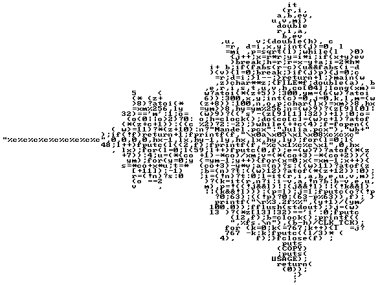### Small mandelbrot set in C!

• 5 Replies
• 292 Views

0 Members and 1 Guest are viewing this topic.

####galac#### Small mandelbrot set in C!

« on: March 02, 2019, 11:21:34 PM »
Hello, I was reading IOCCC submissions when I had the idea to make a really small Mandelbrot set renderer.

Here it is: 216 bytes
Code: [Select]
main(){float t,x,y,a,b;int i=0;while(++i<120000){i%100==1?t=x=y=0,a=i/100%60/20.f-2,b=i/6000/10.f-1:0;t=x,x=x*x-y*y+a,y=2*t*y+b;x*x+y*y>4?t=i=i-i%100+100:0;i%100==0?printf(t==i?" ":"*"):0;i%6000==0?printf("\n"):0;}}
I made a similar version that has gray scale output based on ASCII: 247 bytes
Code: [Select]
main(){float t,x,y,a,b;int i=0;while(++i<120000){i%100==1?t=x=y=0,a=i/100%60/20.f-2,b=i/6000/10.f-1:0;t=x,x=x*x-y*y+a,y=2*t*y+b;x*x+y*y>4?t=i,i=i-i%100+100:0;i%100==0?printf("%c"," #@XMW*N&K"[((int)t%100*30)/100%10]):0;i%6000==0?printf("\n"):0;}}
gcc should be able to compile them.

####marcm200#### Re: Small mandelbrot set in C!

« Reply #1 on: March 03, 2019, 10:10:43 AM »
Very funny idea! I thought I give it a try myself in C++ and ASCII output and came down to 243 bytes source code (checked it with the very old gcc version 3.4.3)
Code: [Select]
#include"stdio.h" main(){double r,i,x,y,t;int n,w;for(y=-1.5;y<=1.2;y+=0.027){for(x=-1.5;x<=0.7;x+=0.022){r=x;i=y;n=100;w=1;while(n>0){t=r*r-i*i;i=2*r*i+y;r=t+x;n--;if((r*r+i*i)>=4){printf(".");w=0;break;}}if(w)printf("X");}printf("\n");}}

####galac#### Re: Small mandelbrot set in C!

« Reply #2 on: March 19, 2019, 03:35:23 PM »
Thank to marcm200, after some searching, I reduced the code to reach 165 bytes:
Code: [Select]
main(){float x,y,t,a,b=1,k;for(;b>-1;b-=.1){for(a=-3;a<1;a+=.1){x=y=t=k=0;while(++k<98){t=x,x=x*x-y*y+a,y=2*t*y+b;x*x+y*y>4?k=99:0;}putchar(k>98?46:42);}puts("");}}

If you compile it with g++, you must add '#include<cstdio>' at the beginning.

####quaz0r#### Re: Small mandelbrot set in C!

« Reply #3 on: March 20, 2019, 01:30:42 AM »
i remember one of those code competitions there was a mandelbrot renderer written as a short C code formatted in the shape of the mandelbrot set.  i cant seem to find it now

####3DickUlus

•• 3f
•• Posts: 1223#### Re: Small mandelbrot set in C!

« Reply #4 on: March 20, 2019, 01:57:39 AM »
https://preshing.com/20110926/high-resolution-mandelbrot-in-obfuscated-python/
Code: [Select]
_                                      =   (                                        255,                                      lambda                               V       ,B,c                             :c   and Y(V*V+B,B,  c                               -1)if(abs(V)<6)else               (              2+c-4*abs(V)**-0.4)/i                 )  ;v,      x=1500,1000;C=range(v*x                  );import  struct;P=struct.pack;M,\            j  ='<QIIHHHH',open('M.bmp','wb').writefor X in j('BM'+P(M,v*x*3+26,26,12,v,x,1,24))or C:            i  ,Y=_;j(P('BBB',*(lambda T:(T*80+T**9                  *i-950*T  **99,T*70-880*T**18+701*                 T  **9     ,T*i**(1-T**45*2)))(sum(               [              Y(0,(A%3/3.+X%v+(X/v+                               A/3/3.-x/2)/1j)*2.5                             /x   -2.7,i)**2 for  \                               A       in C                                      [:9]])                                        /9)                                       )   )
...there's the same thing in C but I can't find it right now... edit: but...
https://www.codeproject.com/Articles/2228/Obfuscating-your-Mandelbrot-code
Code: [Select]
#include "stdio.h"main(){    int b=0,c=0,q=60,_=q;for(float i=-20,o,O=0,l=0,j,p;j=O*O,p=l*l,    (!_--|(j+p>4)?fputc(b?q+(_/3):10,(i+=!b,p=j=O=l=0,c++,stdout)),    _=q:l=2*O*l+i/20,O=j-p+o),b=c%q,c<2400;o=-2+b*.05);}
...this is one of the smallest, 186 chars?, mandelbrot generator I've seen.

and then there's The International Obfuscated C Code Contest that has a lot of really weird stuff(edit: of course you've been there)

edit2: ok found a C version...
https://stijnw.home.xs4all.nl/ocmjg/index.html« Last Edit: March 20, 2019, 02:35:07 AM by 3DickUlus »
Resistance is fertile... you will be illuminated!

https://en.wikibooks.org/wiki/Fractals/fragmentarium

####claude#### Re: Small mandelbrot set in C!

« Reply #5 on: May 13, 2019, 03:21:49 AM »
some small C99:
Code: [Select]
#include <math.h>#include <stdio.h>#include <stdlib.h>#include <time.h>typedef int N;typedef double R;typedef unsigned char B;N main(){srand(time(0));R e=3,a=8,r=100,Z=2,D=18,b=18;N w=1920,h=1080;printf("P6\n%d %d\n255\n",w,h);for(N j=0;j<h;++j){R y=(h*0.5-(j+0.5))/r;B p[w];for(N i=0;i<w;++i){R x=(i+0.5-w*0.5)/r;R S=Z*Z-x*x-y*y;R d=S>0?D-sqrt(S):b;N o=floor(fabs(d-a)*e/d*r+rand()/(R)RAND_MAX);if(i-o<0||o==0)for(N c=0;c<3;++c)p[i][c]=rand();else for(N c=0;c<3;++c)p[i][c]=p[i-o][c];}fwrite(&p,w*3,1,stdout);}return 0;}(exercise make this a Mandelbrot instead)

###Similar Topics###### (Mandelbrot / Burning Ship / Mandelbrot / Mandelbrot) Hybrid

Started by claude on Fractal movie gallery

15 Replies
717 ViewsFebruary 06, 2018, 04:56:54 AM
by claude###### Mandelbrot Burning Ship Mandelbrot Mandelbrot hybrid

Started by claude on Fractal Image Gallery

0 Replies
229 ViewsJanuary 16, 2018, 11:30:30 PM
by claude###### Mandelbrot Burning Ship Mandelbrot Mandelbrot hybrid 2

Started by claude on Fractal Image Gallery

0 Replies
211 ViewsJanuary 17, 2018, 12:10:56 AM
by claude###### Mandelbrot Burning Ship Mandelbrot Mandelbrot hybrid 3

Started by claude on Fractal Image Gallery

0 Replies
248 ViewsJanuary 17, 2018, 12:26:38 AM
by claude###### Mandelbrot 1e-174

Started by therror on Fractal movie gallery

1 Replies
196 ViewsJanuary 02, 2018, 12:52:47 PM
by quaz0r